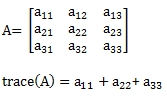# SQL Server matrix trace

MTRACEN_q

Updated: 30 September 2013

Use MTRACEN_q to calculate the trace of a matrix in 3rd normal form. The trace of a matrix (A) is the sum of the diagonal elements. For example:Syntax
SELECT [wctMath].[wct].[MTRACEN_q](
<@Matrix_RangeQuery, nvarchar(max),>)
Arguments
@Matrix_RangeQuery
the SELECT statement, as text, used to determine the matrix to be used in this function. The SELECT statement specifies the row, the column, and the value to be returned from the table or view or can be used to enter the matrix values directly. @Matrix_RangeQuery must always return three columns of data. Data returned from the @Matrix_RangeQuery select must be of the type float or of a type that implicitly converts to float.
Return Types
float
Remarks
·         Use the MTRACEN function for simpler queries.
·         Use MTRACE_q for a de-normalized table.
·         If the matrix is not square then an error will be returned.
·         If the matrix diagonal contains NULL, then NULL will be returned.
·         The function returns an error if the matrix diagonal contains a non-numeric value.
Examples
In this example, we calculate trace from a temporary table containing one matrix.
SET NOCOUNT ON

CREATE TABLE #c(
rn          int,
cn          int,
z           float
)

INSERT INTO #c VALUES (1,1,-78)
INSERT INTO #c VALUES (1,2,-35)
INSERT INTO #c VALUES (1,3,98)
INSERT INTO #c VALUES (1,4,-65)
INSERT INTO #c VALUES (1,5,49)
INSERT INTO #c VALUES (2,1,48)
INSERT INTO #c VALUES (2,2,-53)
INSERT INTO #c VALUES (2,3,-1)
INSERT INTO #c VALUES (2,4,-18)
INSERT INTO #c VALUES (2,5,-12)
INSERT INTO #c VALUES (3,1,35)
INSERT INTO #c VALUES (3,2,-70)
INSERT INTO #c VALUES (3,3,-77)
INSERT INTO #c VALUES (3,4,44)
INSERT INTO #c VALUES (3,5,73)
INSERT INTO #c VALUES (4,1,37)
INSERT INTO #c VALUES (4,2,-75)
INSERT INTO #c VALUES (4,3,-13)
INSERT INTO #c VALUES (4,4,-55)
INSERT INTO #c VALUES (4,5,97)
INSERT INTO #c VALUES (5,1,-56)
INSERT INTO #c VALUES (5,2,36)
INSERT INTO #c VALUES (5,3,84)
INSERT INTO #c VALUES (5,4,-34)
INSERT INTO #c VALUES (5,5,52)

SELECT wct.MTRACEN_q('SELECT rn,cn,z FROM #c') as TRACE

DROP TABLE #c

This produces the following result.
TRACE
----------------------
-211

Using the same data, we can calculate the trace directly from the derived table.
SELECT wct.MTRACEN_q('SELECT rn,cn,z FROM (VALUES
(1,1,-78),
(1,2,-35),
(1,3,98),
(1,4,-65),
(1,5,49),
(2,1,48),
(2,2,-53),
(2,3,-1),
(2,4,-18),
(2,5,-12),
(3,1,35),
(3,2,-70),
(3,3,-77),
(3,4,44),
(3,5,73),
(4,1,37),
(4,2,-75),
(4,3,-13),
(4,4,-55),
(4,5,97),
(5,1,-56),
(5,2,36),
(5,3,84),
(5,4,-34),
(5,5,52)
)n(rn,cn,z)
') as TRACE

This produces the following result.
TRACE
----------------------
-211

Let's put several matrices into a table and calculate the trace for each.
SET NOCOUNT ON

CREATE TABLE #c(
Matrix      int,
rn          int,
cn          int,
z           float,
PRIMARY KEY (Matrix, rn,cn)
)

INSERT INTO #c VALUES (500,1,1,88)
INSERT INTO #c VALUES (500,1,2,32)
INSERT INTO #c VALUES (500,1,3,-19)
INSERT INTO #c VALUES (500,2,1,-14)
INSERT INTO #c VALUES (500,2,2,-7)
INSERT INTO #c VALUES (500,2,3,-87)
INSERT INTO #c VALUES (500,3,1,-99)
INSERT INTO #c VALUES (500,3,2,-43)
INSERT INTO #c VALUES (500,3,3,30)
INSERT INTO #c VALUES (501,1,1,-10)
INSERT INTO #c VALUES (501,1,2,-69)
INSERT INTO #c VALUES (501,1,3,25)
INSERT INTO #c VALUES (501,2,1,-94)
INSERT INTO #c VALUES (501,2,2,83)
INSERT INTO #c VALUES (501,2,3,-40)
INSERT INTO #c VALUES (501,3,1,0)
INSERT INTO #c VALUES (501,3,2,-1)
INSERT INTO #c VALUES (501,3,3,20)
INSERT INTO #c VALUES (502,1,1,-73)
INSERT INTO #c VALUES (502,1,2,-55)
INSERT INTO #c VALUES (502,1,3,1)
INSERT INTO #c VALUES (502,2,1,-84)
INSERT INTO #c VALUES (502,2,2,-41)
INSERT INTO #c VALUES (502,2,3,-92)
INSERT INTO #c VALUES (502,3,1,-70)
INSERT INTO #c VALUES (502,3,2,-69)
INSERT INTO #c VALUES (502,3,3,-70)
INSERT INTO #c VALUES (503,1,1,-41)
INSERT INTO #c VALUES (503,1,2,-90)
INSERT INTO #c VALUES (503,1,3,86)
INSERT INTO #c VALUES (503,2,1,-90)
INSERT INTO #c VALUES (503,2,2,88)
INSERT INTO #c VALUES (503,2,3,24)
INSERT INTO #c VALUES (503,3,1,52)
INSERT INTO #c VALUES (503,3,2,60)
INSERT INTO #c VALUES (503,3,3,-37)

SELECT Matrix
,wct.MTRACEN_q('SELECT rn,cn,z FROM #c WHERE Matrix = ' + cast(n.Matrix as varchar(max))) as TRACE
FROM (SELECT DISTINCT Matrix from #c)n

DROP TABLE #c

This produces the following result.
Matrix                  TRACE
----------- ----------------------
500                    111
501                     93
502                   -184
503                     10

### SupportCopyright 2008-2021 Westclintech LLC         Privacy Policy        Terms of Service